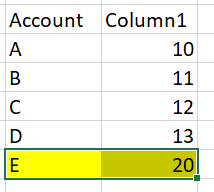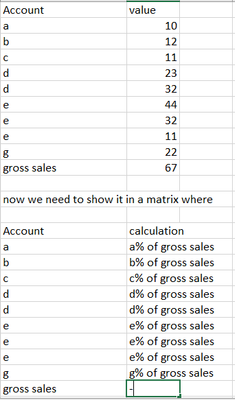cancel
Showing results for
Did you mean:New Member

## Divide values from a column by specifc values of same column and display in matrix

I have a data looks like belowIts a matrix where calculation is like
(sum of all values of column1 /sum of E)*100

so it has be for each row of account column.

5 REPLIES 5New Member

.i refraim my query
top most view is data and then matrix view.
To conclude.

all sum of rows must be divide by same value gross sales *100Solution SageNew Member

gross sales is same as other values as account in that columnSolution Sage
Measure =
Var _sumall = CALCULATE(sum('Table'[Value]),ALLSELECTED('Table'))
Var _currentvalue = sumx('Table','Table'[Value])
RETURN _currentvalue/_sumallSolution Sage
`````` = DIVIDE(
CALCULATE(sum('table'[column1], REMOVEFILTERS(),
sum('table'[column1])``````